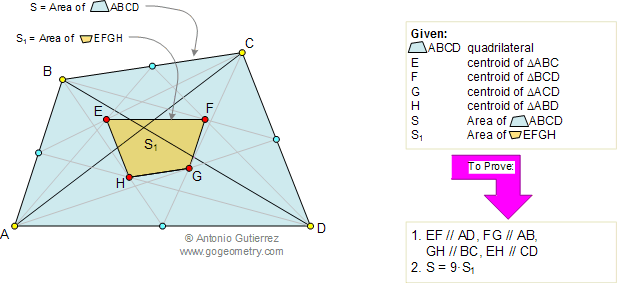Problem 98. Quadrilateral Areas, Centroids, Similarity. High School, College

 In the diagram below, given a quadrilateral ABCD of area S. E, F, G, and H are the centroids of the triangles ABC, BCD, ACD, and ABD respectively. S1 is the area of the quadrilateral EFGH. Prove that (1) EF, FG, GH, and EH are parallel to AD, AB, BC, and CD respectively; (2) S = 9S1. View or post a solution. .FACTS AND HINTS:

1. CENTROID:
The geometric centroid (center of mass) or barycenter of the polygon vertices of a triangle is the point G which is also the intersection of the triangle's three triangle medians.

Proposition: The centroid divides each of the medians in the ratio 2:12. PROPORTIONAL SEGMENTS:

Proposition 1: If a line is parallel to one side of a triangle, then it divides the other two sides proportionally.

Proposition 2: If a line divides two sides of a triangle proportionally, it is parallel to the third side. (Converse of -proposition 1.)

3. SIMILAR POLYGONS - Ratio of Areas:

Proposition: If two polygons are similar, the ratio of their areas is equal to the square of the ratio of their corresponding sides.

Home | Geometry | Search | Problems | Similarity | Wolfram Demonstrations Project | Parallelograms | All Problems | 91-100 | Visual Index | Email | View or post a solution | by Antonio Gutierrez